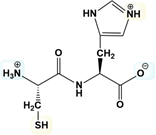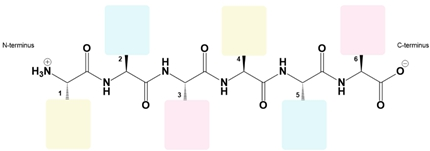## Biochemistry

Learn the toughest concepts covered in Biochemistry with step-by-step video tutorials and practice problems by world-class tutors

4. Protein Structure

# Isoelectric Point of a Peptide

1
concept

## Isoelectric Point of a Peptide13m
Play a video:
2
Problem

Estimate the isoelectric point of the following dipeptide in the figure.3
Problem

Calculate the approximate pI of the peptide: C-G-E-K.

4
Problem

Draw in the R-groups for the following peptide & calculate the pI: ATLDAG.